﻿ Bending channel with a porous media region (explicit method) - XSim

# Bending channel with a porous media region (explicit method)

Update: November 26, 2018
OpenFOAM 4.x

$FOAM_TUTORIALS/compressible/rhoPorousSimpleFoam/angledDuctExplicit ## Summary We calculate a flow with a porous material that models a filter in the middle of the flow path. The fluid flows in from the region "inlet" (end face of blue part) at a mass flow rate of 0.1 kg/s, passes through the filter (red part), and flows out from the region "outlet" (end face of green part).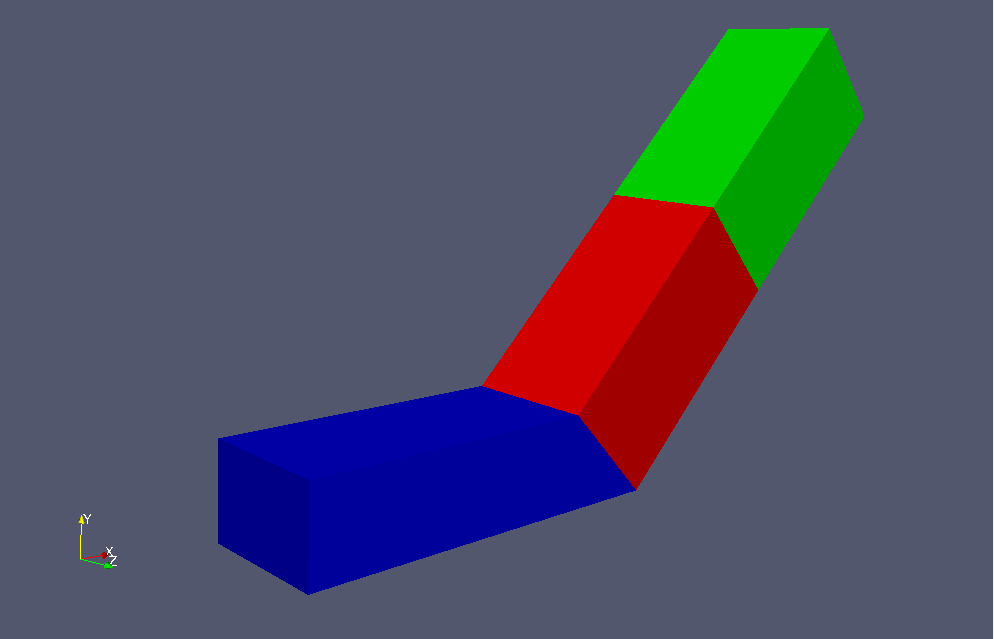Model geometry Porous media depends on the Darcy-Forchheimer law, and for a flow velocity ui (i=x, y, z) in direction i, a generation term Si (pressure drop) in the opposite direction of flow is added to the Navier-Stokes equations. Here, μ is the viscosity coefficient and ρ is the density. The parameters that determine the properties of the porous media, Dij, F, the direction of the properties, and the region in which the porous media, are specified in the file constant/porosityProperties as follows. porosity1 { type DarcyForchheimer; active yes; cellZone porosity; DarcyForchheimerCoeffs { d (5e7 -1000 -1000); f (0 0 0); coordinateSystem { type cartesian; origin (0 0 0); coordinateRotation { type axesRotation; e1 (0.70710678 0.70710678 0); e2 (0 0 1); } } } }  If you do not specify "nUCorrectors" in "SIMPLE" in the file system/fvSolution (or specify 0), the calculation will be performed in an explicit way instead of an implicit way. The standard k-ε model is used for the turbulence model. The meshes are as follows, and the number of mesh is 22000.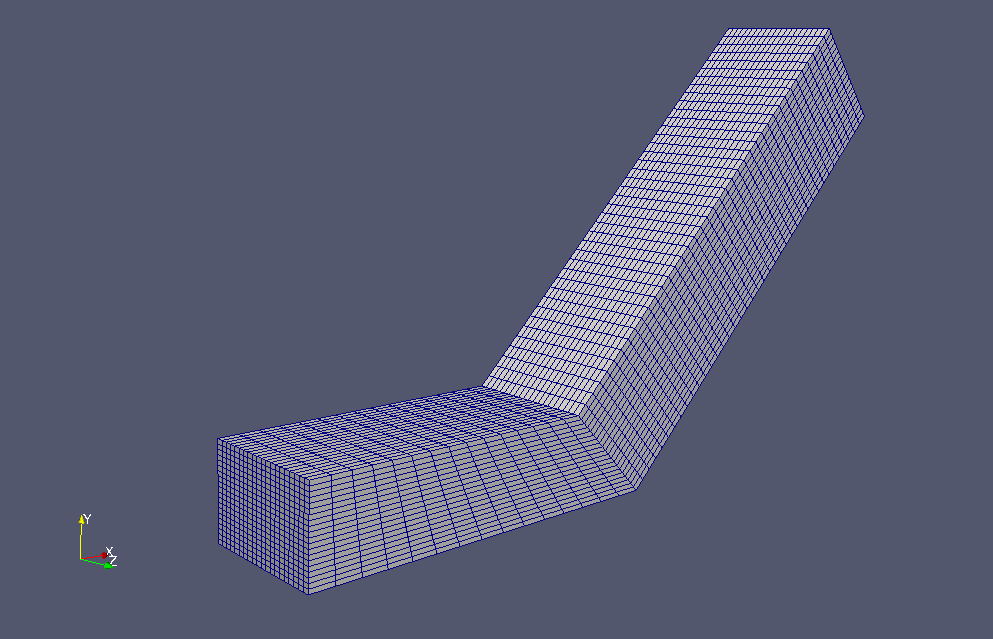Meshes If you want to visualize turbulent energy and turbulent dissipation rate, check "k" and "epsilon" in the "Properties" tab in ParaView.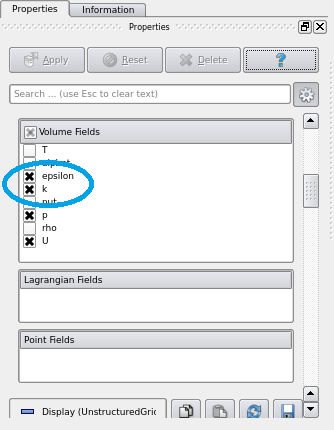Check "k" and "epsilon" in "Properties" tab The calculation result is as follows.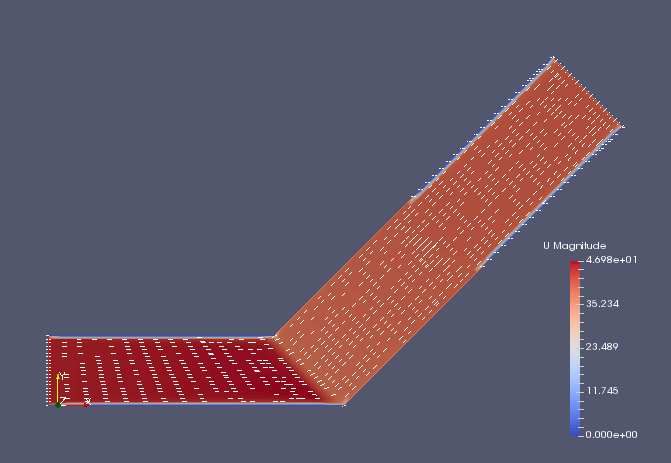Flow velocity (U)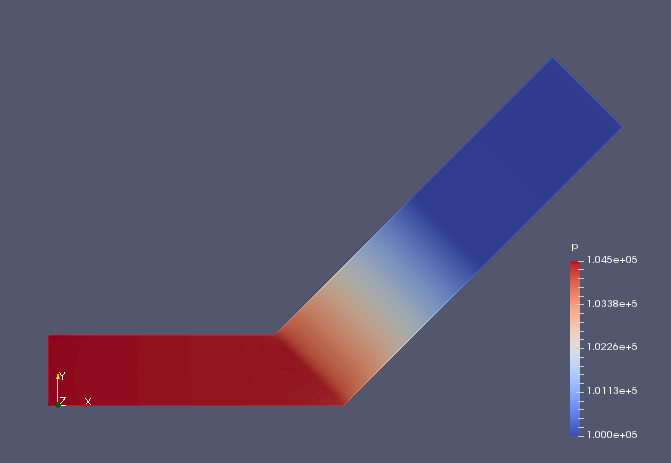Pressure (p)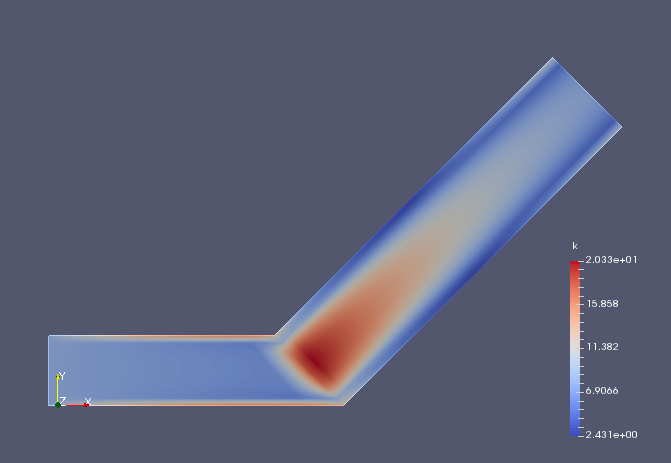Turbulent energy (k)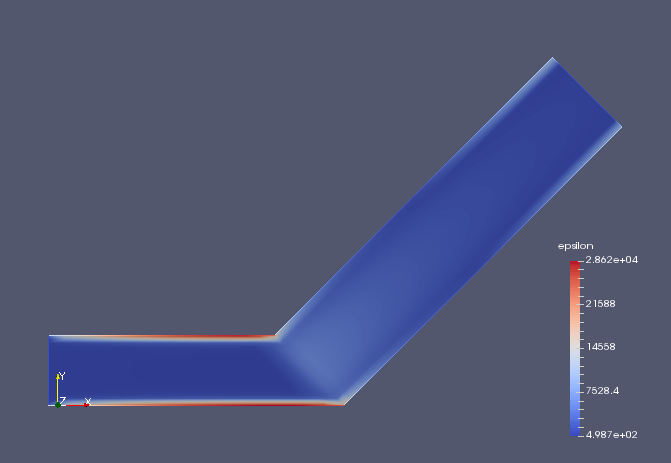Turbulent dissipation rate (epsilon) We can see that the pressure are greatly reduced in the porous media part. ## Commands cp -r$FOAM_TUTORIALS/compressible/rhoPorousSimpleFoam/angledDuctImplicit angledDuctImplicit
cp -r \$FOAM_TUTORIALS/compressible/rhoPorousSimpleFoam/angledDuctExplicit angledDuctExplicit
cd angledDuctExplicit

m4 system/blockMeshDict.m4 > system/blockMeshDict
blockMesh
rhoPorousSimpleFoam

paraFoam

In this tutorial, we use the "0" and "constant" directories in the tutorial "Bending channels with a porous media region (implicit method)". Therefore, the case "angledDuctImplicit" must be placed in the same directory as the case "angledDuctExplicit".

## Calculation time

22.67 seconds *Single, Inter(R) Core(TM) i7-8700 CPU @ 3.20GHz 3.19GHz